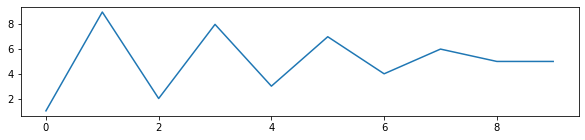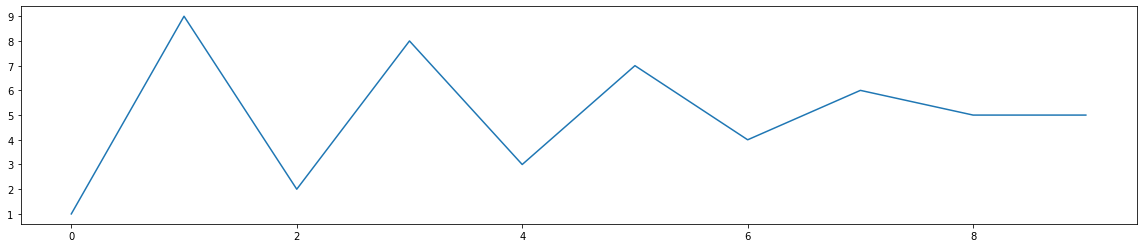Question #609

# In Python, how do you change the size of figures drawn with Matplotlib?

Merged questions

The simplest way to change the size of an existing Matplotlib figure is to use the method ﻿`set_size_inches()`:

``````import matplotlib.pyplot as plt
plt.plot ([1,9,2,8,3,7,4,6,5,5])

# Set figure size in inches
fig = plt.gcf()
fig.set_size_inches(20, 4)``````

The above code should display something like:7 events in history
Question by Alphonsio 01/11/2021 at 03:57:57 PM
In Python, how do you change the size of an already created figure drawn with Matplotlib?
Answer by Alphonsio 01/11/2021 at 03:57:14 PM

The simplest way to change the size of an existing Matplotlib figure is to use the method ﻿`set_size_inches()`:

``````import matplotlib.pyplot as plt
plt.plot ([1,9,2,8,3,7,4,6,5,5])

# Set figure size in inches
fig = plt.gcf()
fig.set_size_inches(20, 4)``````

The above code should display something like:Answer by Alphonsio 01/11/2021 at 03:56:25 PM

The simplest way to change the size of an existing Matplotlib figure is to use set_size_inches():

``````import matplotlib.pyplot as plt
plt.plot ([1,9,2,8,3,7,4,6,5,5])

# Set figure size in inches
fig = plt.gcf()
fig.set_size_inches(20, 4)``````

The above code should display something like:Answer by Alphonsio 01/11/2021 at 03:56:07 PM

The simplest way to change the size of an existing Matplotlib figure is to use set_size_inches():

``````import matplotlib.pyplot as plt
plt.plot ([1,9,2,8,3,7,4,6,5,5])

# Set figure size in inches
fig = plt.gcf()
fig.set_size_inches(20, 4)``````

The above code should display something like:Answer by Alphonsio 01/10/2021 at 01:30:34 PM

The simplest way to change the size of a Matplotlib figure is to set the ﻿rcParams as on the following example :

``````import matplotlib.pyplot as plt
# Set figure size in inches
plt.rcParams["figure.figsize"] = (8,6)
plt.plot (data)``````

Answer by Alphonsio 01/10/2021 at 01:15:25 PM

The simplest way to change the size of a Matplotlib figure is to set the ﻿rcParams as on the following example :

``````import matplotlib.pyplot as plt
plt.rcParams["figure.figsize"] = (8,6)
plt.plot (data)``````

Question by Alphonsio 01/10/2021 at 01:11:05 PM
In Python, how do you change the size of figures drawn with Matplotlib?

Icons proudly provided by Friconix.